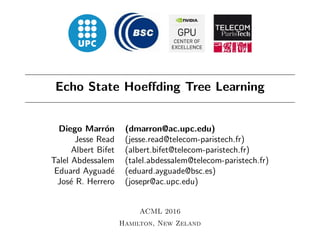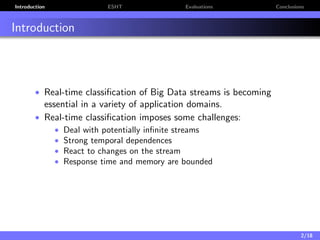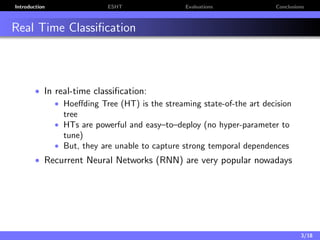Successfully reported this slideshow.

# Echo State Hoeffding Tree Learning×

# Echo State Hoeffding Tree Learning

In this work we propose a novel architecture for real-time classification based on the combination of a Reservoir and a decision tree. This combination makes classification fast, reduces the number of hyper-parameters and keeps the good temporal properties of recurrent neural networks.
The capabilities of the proposed architecture to learn some typical string-based functions with strong temporal dependences are evaluated in the paper. The paper shows how the new architecture is able to incrementally learn these functions in real-time with fast adaptation to unknown sequences and analyzes the influence of the reduced number of hyper-parameters in the behaviour of the proposed solution.

In this work we propose a novel architecture for real-time classification based on the combination of a Reservoir and a decision tree. This combination makes classification fast, reduces the number of hyper-parameters and keeps the good temporal properties of recurrent neural networks.
The capabilities of the proposed architecture to learn some typical string-based functions with strong temporal dependences are evaluated in the paper. The paper shows how the new architecture is able to incrementally learn these functions in real-time with fast adaptation to unknown sequences and analyzes the influence of the reduced number of hyper-parameters in the behaviour of the proposed solution.

### Echo State Hoeffding Tree Learning

1. 1. Echo State Hoeﬀding Tree Learning Diego Marr´on (dmarron@ac.upc.edu) Jesse Read (jesse.read@telecom-paristech.fr) Albert Bifet (albert.bifet@telecom-paristech.fr) Talel Abdessalem (talel.abdessalem@telecom-paristech.fr) Eduard Ayguad´e (eduard.ayguade@bsc.es) Jos´e R. Herrero (josepr@ac.upc.edu) ACML 2016 Hamilton, New Zeland
2. 2. Introduction ESHT Evaluations Conclusions Introduction • Real-time classiﬁcation of Big Data streams is becoming essential in a variety of application domains. • Real-time classiﬁcation imposes some challenges: • Deal with potentially inﬁnite streams • Strong temporal dependences • React to changes on the stream • Response time and memory are bounded 2/18
3. 3. Introduction ESHT Evaluations Conclusions Real Time Classiﬁcation • In real-time classiﬁcation: • Hoeﬀding Tree (HT) is the streaming state-of-the art decision tree • HTs are powerful and easy–to–deploy (no hyper-parameter to tune) • But, they are unable to capture strong temporal dependences • Recurrent Neural Networks (RNN) are very popular nowadays 3/18
4. 4. Introduction ESHT Evaluations Conclusions Recurrent Neural Networks • Recurrent Neural Networks (RNNs) are the state-of-the-art in handwriting recognition, speech recognition, natural language processing among others • They are able to capture time dependences • But their use for data streams is not straight forward • Very sensitive to hyper-parameters conﬁguration • Training requires many iterations over data... • ...and large amount of time 4/18
5. 5. Introduction ESHT Evaluations Conclusions RNN: Echo State Network • A type of Recurrent Neural Network • Echo State Layer (ESL): • Dynamics only driven by the input • Requires very few computations • Easy to understand hyper-parameters • Can capture time dependences • ESN also requires the hyper-parameters needed by the NN • Gradient Descent methods have slow convergence 5/18
6. 6. Introduction ESHT Evaluations Conclusions Contribution • Objective: • Need to model the evolution of the stream over time • Reduce number of hyper-parameters • Reduce amount of samples needed to learn • In this work we present the ESHT: • Combination of HT + ESL • To learn temporal dependences in data streams in real-time • Requires less hyper-parameters than the ESN 6/18
7. 7. Introduction ESHT Evaluations Conclusions ESHT • Echo State Layer (ESL): • Only needs two hyper-parameters: • Alpha (α): weights events in X(n) importance over new ones • Density: Wres is a sparse matrix with given density • Encodes time-dependences • FIMT-DD: Hoeﬀding tree for regression • Works out-of-the-box: no hyper-parameters tuning 7/18
8. 8. Introduction ESHT Evaluations Conclusions ESHT: Evaluation Methodology • We propose the ESHT to learn character-stream functions: • Counter (skipped in this presentation) • lastIndexOf • emailFilter • lastIndexOf Evaluation: • Study the eﬀects of hyper-parameters: α and density • Alpha (α): weights events in X(n) importance over new ones • Density: Wres is a sparse matrix with given density • Use 1,000 neurons on the ESL • emailFilter evaluation: • We focus on the speed of learning • Use outcomes from previous evaluations to conﬁgure the ESHT for this task • Metrics: • Cumulative loss • We consider an error if |yt − ˆy| >= 0.5 8/18
9. 9. Introduction ESHT Evaluations Conclusions Input format • Input is a vector of ﬂoats • Number of attributes = number of input symbols • Attribute representing current symbol set to 0.5 • Other attributes are set to zero 9/18
10. 10. Introduction ESHT Evaluations Conclusions LastIndexOf • Counts the number of time steps since the current symbol was last observed • Input stream is randomly generated • We 2,3 and 4 symbols 10/18
11. 11. Introduction ESHT Evaluations Conclusions LastIndexOf: Vector vs Scalar Input • Vector input improves accuracy in all cases • Specially with 4 symbols 0.1 0.2 0.3 0.4 0.5 0.6 0.7 0.8 0.9 1 0.1 0.2 0.3 0.4 0.5 0.6 0.7 0.8 0.9 1 α Accuracy(%) 2symbols density=0.4 2symbols-vec density=0.4 3symbols density=0.4 3symbols-vec density=0.4 4symbols density=0.4 4symbols-vec density=0.4 11/18
12. 12. Introduction ESHT Evaluations Conclusions LastIndexOf: Alpha and Density vs Accuracy • Lower values of alpha (α) have low accuracy • There is no clear correlation between accuracy and density 0.1 0.2 0.3 0.4 0.5 0.6 0.7 0.8 0.9 1.0 0.1 0.2 0.3 0.4 0.5 0.6 0.7 0.8 0.9 1 Alpha (α) Accuracy(%) 2symbols density=0.1 2symbols density=0.4 3symbols density=0.1 3symbols density=0.4 4symbols density=0.1 4symbols density=0.4 0.1 0.2 0.3 0.4 0.5 0.6 0.7 0.8 0.9 1.0 0.3 0.4 0.5 0.6 0.7 0.8 Density Accuracy(%) α=0.2 α=0.3 α=0.4 α=0.5 α=0.6 α=0.7 α=0.8 α=0.9 α=1.0 12/18
13. 13. Introduction ESHT Evaluations Conclusions EmailFilter • ESHT conﬁguration: • ESL: 4,000 neurons • α = 1.0 and density = 0.1 • Outputs the length on the next space character • Dataset: 20 newsgroups dataset • Extracted 590 characters and repeated them 8 times • To reduce the memory usage we used an input vector of 4 symbols 13/18
14. 14. Introduction ESHT Evaluations Conclusions EmailFilter: Recurrence vs Non Recurrence • Non-recurrent methods (FIMT-DD and NN) fail to capture temporal dependences • NN defaults to majority class Algorithm Density α Learning rate Loss Accuracy (%) FIMT-DD - - - 4,119.7 91.61 NN - - 0.8 2,760 97.80 ESN1 0.2 1.0 0.1 1,032 98.47 ESN2 0.7 1.0 0.1 850 98.47 ESHT 0.1 1.0 - 180 99.75 14/18
15. 15. Introduction ESHT Evaluations Conclusions EmailFilter: ESN vs ESHT • After 500 samples the ESHT loss is close to 0 (and 0 loss after the 1,000 samples) 0 1,000 2,000 3,000 4,000 0 200 400 600 800 1,000 1,200 500 # Samples CummulativeLoss ESN1 ESN2 ESHT 15/18
16. 16. Introduction ESHT Evaluations Conclusions Conclusions and Future Work • Conclusions: • We presented the ESHT to learn temporal dependences in data streams in real-time • The ESHT requires less hyper-parameters than the ESN • Our proof-of-concept implementation is able to learn faster than an ESN (Most of them at ﬁrst shot) • Future Work: • We are currently reimplementing our prototype so we can test larger input sequences • We need to study the eﬀects of the initial state vanishing in large sequences 16/18
17. 17. Thank you
18. 18. Echo State Hoeﬀding Tree Learning Diego Marr´on (dmarron@ac.upc.edu) Jesse Read (jesse.read@telecom-paristech.fr) Albert Bifet (albert.bifet@telecom-paristech.fr) Talel Abdessalem (talel.abdessalem@telecom-paristech.fr) Eduard Ayguad´e (eduard.ayguade@bsc.es) Jos´e R. Herrero (josepr@ac.upc.edu) ACML 2016 Hamilton, New Zeland
19. 19. ESHT: Module Architecture • In each evaluation we use the following architecture • Label generator implements the function to be learnt 1/0
20. 20. Counter: Introduction • Stream of zeros and ones randomly generated • Input is a scalar • Two variants: • Option1: Outputs cumulative count • Option2: Outputs total count on the next zero 2/0
21. 21. Counter: Cumulative Loss • After 200 samples the loss is stable 0 200 400 600 800 1,000 0 10 20 30 # Samples CummulativeLoss Op1(density=0.3,α=1.0) Op1(density=1.0,α=0.7) Op2(density=0.8,α=1.0) Op2(density=0.8,α=0.7) 3/0
22. 22. Counter: Alpha and Density vs Accuracy 0.1 0.2 0.3 0.4 0.5 0.6 0.7 0.8 0.9 1.0 0.5 0.6 0.7 0.8 0.9 1 Alpha (α) Accuracy(%) 0.1 0.2 0.3 0.4 0.5 0.6 0.7 0.8 0.9 1.0 0.5 0.6 0.7 0.8 0.9 1 Density (%) Accuracy(%) 4/0
23. 23. EmailFilter: ASCII to 4 symbols Table ASCII Domain 4-Symbols Domain Original Symbols Target Symbol Target Symbol Index [t n r]+ Single space 0 [a-zA-Z0-9] x 1 @ @ 2 . . 3 5/0